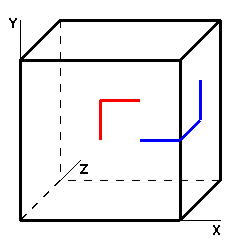### Problem Statement

You are given a cube with side length S and vertices at (0,0,0), (0,0,S), (0,S,0), (S,0,0), (S,S,0), (S,0,S), (0,S,S) and (S,S,S). You are also given two points (x1,y1,z1) and (x2,y2,z2) on the faces of the cube. The distance between the points on the cube is defined as the minimal length of a polygonal curve which connects the two points. Each segment of that polygonal curve must be on a face of the cube, parallel to one of the cube's edges. The following image shows two pairs of points, connected with the shortest polygonal curves.Return the distance between the given points.

### Definition

 Class: DistanceOnCube Method: calculate Parameters: int, int, int, int, int, int, int Returns: int Method signature: int calculate(int S, int x1, int y1, int z1, int x2, int y2, int z2) (be sure your method is public)

### Notes

-A polygonal curve is a connected sequence of line segments. It contains a sequence of vertices A1, ..., An, where each pair of consecutive vertices is connected by a line segment.

### Constraints

-S will be between 1 and 100, inclusive.
-x1, y1, z1, x2, y2, z2 will all be between 0 and S, inclusive.
-Points (x1,y1,z1) and (x2,y2,z2) will belong to the faces of the cube.

### Examples

0)

 `4` `2` `2` `0` `3` `3` `0`
`Returns: 2`
 The points are placed on the same face of the cube, so the shortest polygonal curve that connects them consists of two segments, for example, (2,2,0) - (2,3,0) - (3,3,0) (the red curve on the image).
1)

 `4` `3` `2` `0` `4` `3` `2`
`Returns: 4`
 The points are placed on adjacent faces of the cube (the blue curve on the image).
2)

 `4` `1` `1` `0` `1` `1` `4`
`Returns: 6`
 The points are placed on opposite faces of the cube.
3)

 `4` `2` `3` `0` `2` `3` `0`
`Returns: 0`
 The points coincide.
4)

 `87` `87` `10` `3` `44` `11` `0`
`Returns: 47`
5)

 `49` `0` `13` `43` `49` `10` `45`
`Returns: 62`
6)

 `48` `13` `0` `5` `8` `48` `1`
`Returns: 59`
7)

 `77` `17` `20` `77` `2` `35` `0`
`Returns: 111`

#### Problem url:

http://www.topcoder.com/stat?c=problem_statement&pm=10794

#### Problem stats url:

http://www.topcoder.com/tc?module=ProblemDetail&rd=14225&pm=10794

Nickolas

#### Testers:

PabloGilberto , ivan_metelsky , StevieT

Geometry, Search